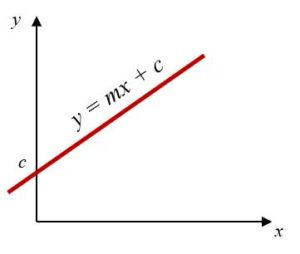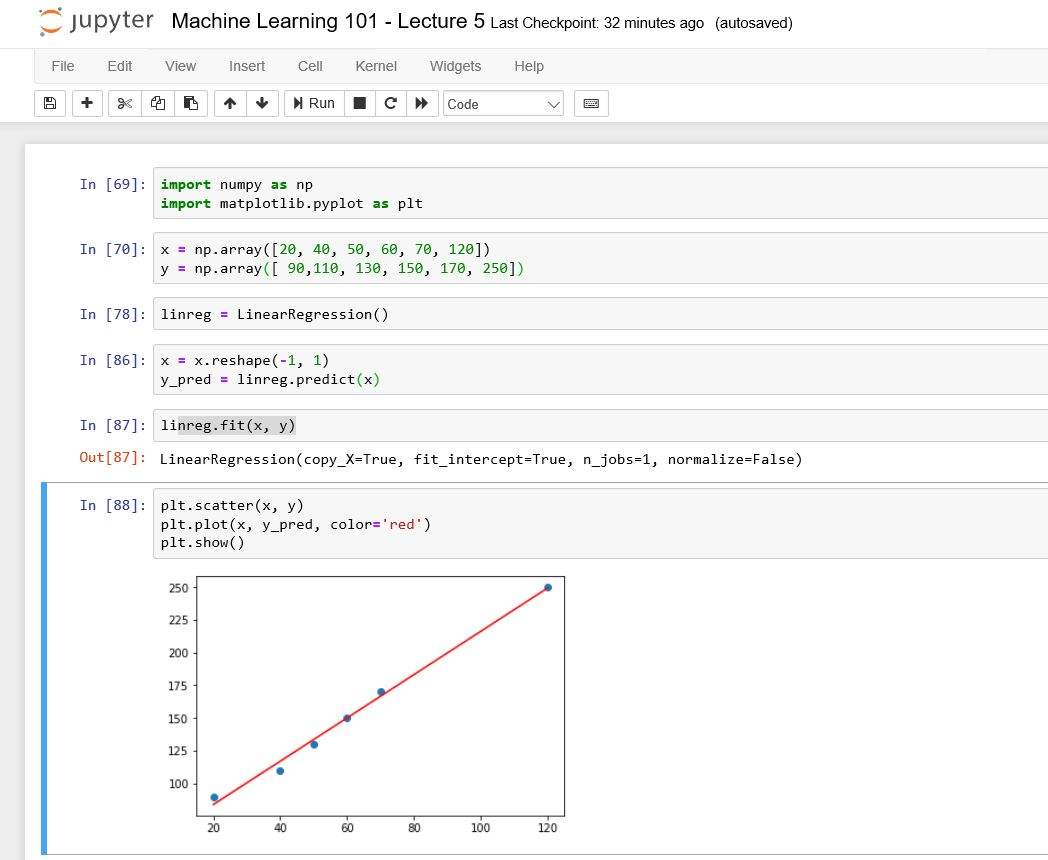# Machine Learning 101 – Equation for a Line and Regression Line

Let’s go back to the regression problem we solved in Lecture 4. We are given a dataset.  You need to find the relationship between the two variables.

The record is given below:

 Spending (x) Profit (t) 40 90 50 110 60 130 70 150 80 170 120 250

Note that we’ve removed the year column. I have also modified the data.

Also, we would call the Spendings column, x and the Profit column y.

Here we can represent this table as:

x = {40, 50, 60, 70, 80, 120}

t = {90, 110, 130, 250, 170, 240}

x is a column vector made up of 6 elements. This can be written also as:

x = {x1, x2, . . . , xn}T where N = 6

Similarly, for y, we have

t = {t1, t2, . . . , tN}T where N = 6

Our goal is to used this data set (training data) to make prediction. So if we have a new value of x, let’s say xi, what would be the corresponding ti.

One way to achieve this is to use a method called curve fitting(or polynomial curve fitting).

Before we discuss curve fitting, let’s review Equation of a Line.

#### Review of Equation for a Line

If you did some mathematics, then you will remember that every line has an equation.

The equation for a line has the general form:

y = mx + c or

y  = c + mx

where;

m is the slope of the line and

c is the intercept of the line on the y axis

This is the generic relationship between x and y that can be plotted on a straight line

This is illustrated in the figure below.This means that the relationship between the two variables is given by the equation of the line.

So if we can find the values of m and c, then we just plug it into the equation.

Now let’s rewrite this equation in a more Machine Learning way

y = ß0 + ß1x

This then means that regression is simply a problem of finding ß0 and ß1 which we call the regression coefficient

#### Practical: Using Python to find Regression Coefficients

Let’s do a little practical. We would find the regression coefficients of using Python.

The Jupyter Notebook screenshot is given below:In the next Lecture, we would continue with Polynomial Curve Equation.#### kindsonthegenius

Kindson Munonye is currently completing his doctoral program in Software Engineering in Budapest University of Technology and Economics

View all posts by kindsonthegenius →